### Stochastic Curtailment

Lan, Simon, and Halperin (1982) introduce stochastic curtailment to stop a trial if, given current data, it is likely to predict the outcome of the trial with high probability. That is, a trial can be stopped to reject the null hypothesisif, given current data in the analyses, the conditional probability of rejectingunderat the end of the trial is greater than, where the constantshould be between 0.5 and 1 and values of 0.8 or 0.9 are recommended (Jennison and Turnbull, 2000, p. 206). Similarly, a trial can be stopped to accept the null hypothesisif, given current data in the analyses, the conditional probability of rejectingunder the alternative hypothesis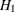at the end of the trial is less than.

The following two approaches for stochastic curtailment are available in the SEQTEST procedures: conditional power approach and predictive power approach. For each approach, the derived group sequential test is used as the reference test for rejection.

#### Conditional Power Approach

In the SEQTEST procedure, you can compute two types of conditional power as described in the following sections:

##### TYPE=ALLSTAGES

The default TYPE=ALLSTAGES suboption in the CONDPOWER and PLOT=CONDPOWER options computes the conditional power at an interim stage k as the total probability of rejecting the null hypothesis at all future stages given the observed statistic (Zhu, Ni, and Yao, 2011, pp. 131–132).

For a one-sided test with an upper alternative, the conditional power at an interim stage k is given by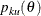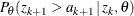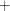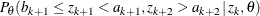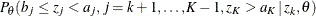whereis the observed statistic andis the hypothetical reference. The conditional power for a one-sided test with a lower alternative is similarly derived.

For a two-sided test, the conditional power for the upper alternative is given by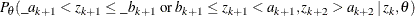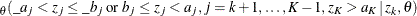The conditional power for the lower alternative is similarly derived.

##### TYPE=FINALSTAGE

The TYPE=FINALSTAGE suboption in the CONDPOWER and PLOT=CONDPOWER options computes the conditional power at an interim stage k as the probability that the test statistic at the final stage (stage K) would exceed the rejection critical value given the observed statistic (Jennison and Turnbull, 2000, p. 207).

The conditional distribution of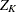given the observed statisticat the kth stage and the hypothetical referenceis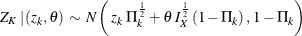where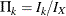is the fraction of information at the kth stage.

The power for the upper alternative,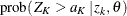, is then given by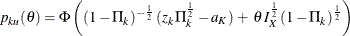where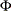is the cumulative distribution function of the standardized Z statistic and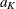is the upper critical value at the final stage.

Similarly, the power for the lower alternative,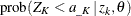, is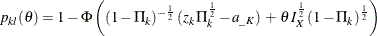where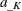is the lower critical value at the final stage.

If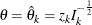, the maximum likelihood estimate at stage k, the powers for the upper and lower alternatives can be simplified: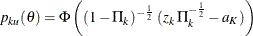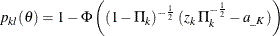If there exist interim stages between the kth stage and the final stage,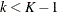, the conditional power computed with TYPE=FINALSTAGE is not the conditional probability to reject the null hypothesis. In this case, you can set the next stage as the final stage, and the conditional power is the conditional probability of rejecting.

A special case of the conditional power is the futility index (Ware, Muller, and Braunwald, 1985). It is 1 minus the conditional power under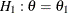: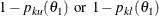That is, it is the probability of accepting the null hypothesis under the alternative hypothesis given current data. A high futility index indicates a small probability of success (rejecting) given the current data.

#### Predictive Power Approach

The conditional power depends on the specified reference, which might be supported by the current data (Jennison and Turnbull, 2000, p. 210). An alternative is to use the predictive power (Herson, 1979), which is a weighted average of the conditional power over values of. Without prior knowledge about, then with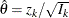, the maximum likelihood estimate at stage k, the posterior distribution for(Jennison and Turnbull, 2000, p. 211) is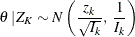Thus, the predictive power at stage k for the upper and lower alternatives can be derived as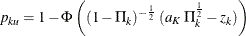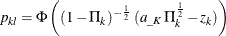whereandare the upper and lower critical values at the final stage.